# AP Board 6th Class Maths Solutions Chapter 10 Practical Geometry Unit Exercise

AP State Syllabus AP Board 6th Class Maths Solutions Chapter 10 Practical Geometry Unit Exercise Textbook Questions and Answers.

## AP State Syllabus 6th Class Maths Solutions 10th Lesson Practical Geometry Unit ExerciseQuestion 1.
Construct a circle with centre X and diameter 10 cm. Sol. Given diameter of circle d = 10 cm
Solution:
We know that radius of circle r = $$\frac{d}{2}=\frac{10}{2}$$ = 5
So, radius of the circle is 5 cm.
Now, draw a circle with radius 5 cm.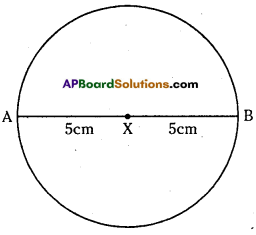Question 2.
Draw four circles of radius 2 cm, 3 cm, 4 cm and 5 cm with the same centre P.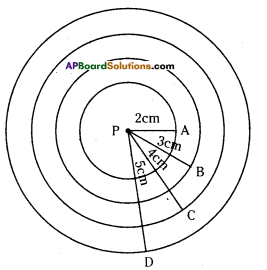Solution:
Radii of four circles are PA = 2cm, PB = 3cm, PC = 4cm and PD = 5cm with the same centre P was constructed.Question 3.
Draw the angles given below using a protractor.
(i) 75°
(ii) 15°
(iii) 105°
Solution:
(i) 75°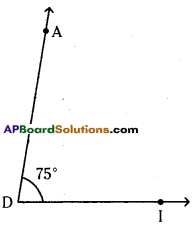Steps of construction :

1. Draw a ray $$\overrightarrow{\mathrm{DI}}$$ with vertex D.
2. Place the centre point of the protractor at D and the line be aligned with DI.
3. Mark a point A at 75°.
4. Join DA. ∠ADI = 75° is formed.
Hence the required angle ∠ADI = 75° is constructed.

ii) 15°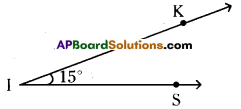∠KIS = 15°
Steps of construction:

1. Draw a ray $$\overrightarrow{\mathrm{IS}}$$ with vertex I.
2. Place the centre point of the protractor at I and the line be aligned with $$\overrightarrow{\mathrm{IS}}$$.
3. Mark a point K at 15°.
4. Join IK.∠KIS = 15° is formed.
Hence the required angle ∠KIS = 15° is constructed.

(iii) 105°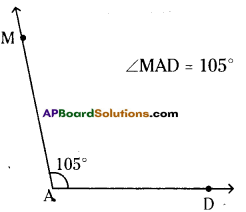Steps of construction:

1. Draw a ray $$\overrightarrow{\mathrm{AD}}$$ with initial point A.
2. Place the centre point of the protractor
at A and the line be aligned with $$\overrightarrow{\mathrm{AD}}$$.
3. Mark a point M at 105°.
4. Join AM.∠MAD = 105° is formed.
Hence the required angle∠MAD = 105° ¡s constructed.Question 4.
Construct ∠ABC = 50° and then draw another angle ∠XYZ equal to ∠ABC without using a protractor.
Solution: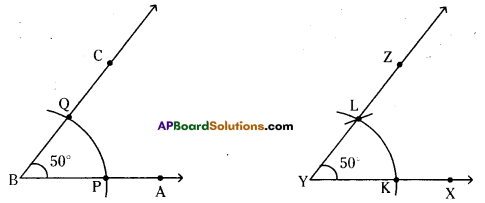Given ∠ABC = 50° and ∠XYZ = 50°.
Steps of construction:

1. Construct ∠ABC = 50°by using protractor.
2. By taking any radius draw arcs from B on AB and BC at P and Q respectively.
3. Draw a ray $$\overrightarrow{\mathrm{YX}}$$ with initial point Y.
4. By taking BP as radius draw an arc on $$\overrightarrow{\mathrm{YX}}$$ from Y which meets at K.
5. Draw arc from Y by taking PQ as radius in the ∠BAC which cuts the previous arc and mark it as L. Now draw $$\overrightarrow{\mathrm{YZ}}$$. So, ∠XYZ = 50° is formed.
Hence ∠XYZ = 50° is constructed which is equal to ∠ABC = 50°.

Question 5.
Construct ∠DEF = 60°. Bisect it, measure each half by using a protractor.
Solution: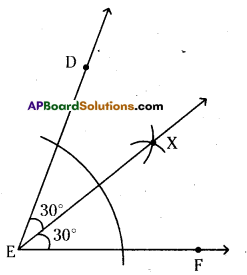Given ∠DEF = 60°
Draw EX as the bisector of ∠DEF.
So, ∠DEX = ∠XEF = $$\frac{\angle \mathrm{DEF}}{2}=\frac{60^{\circ}}{2}$$ = 30°
∴ ∠DEX = ∠XEF = 30°